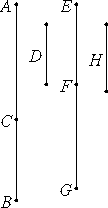# Proposition 49

To find the second binomial line.

Set out two numbers AC and CB such that the sum of them AB has to BC the ratio which a square number has to a square number, but does not have to AC the ratio which a square number has to a square number. Set out a rational straight line D, and let EF be commensurable in length with D, therefore EF is rational.

Let it be contrived then that as the number CA is to AB, so is the square on EFto the square on FG, therefore the square on EF is commensurable with the square on FG. Therefore FG is also rational.Now, since the number CA does not have to AB the ratio which a square number has to a square number, neither does the square on EF have to the square on FG the ratio which a square number has to a square number.

Therefore EF is incommensurable in length with FG. Therefore EF and FG are rational straight lines commensurable in square only. Therefore EG is binomial.

It is next to be proved that it is also a second binomial straight line.

V.7.Cor

Since, inversely, the number BA is to AC as the square on GF is to the square on FE, while BA is greater than AC, therefore the square on GF is greater than the square on FE.

V.19,Cor.

Let the sum of the squares on EF and H equal the square on GF. Then, in conversion, AB is to BC as the square on FG is to the square on H.

But AB has to BC the ratio which a square number has to a square number, therefore the square on FG also has to the square on H the ratio which a square number has to a square number.

X.9

Therefore FG is commensurable in length with H, so that the square on FG is greater than the square on FE by the square on a straight line commensurable with FG.

And FG and FE are rational straight lines commensurable in square only, and EF, the lesser term, is commensurable in length with the rational straight line D set out.

Therefore EG is a second binomial straight line.

Q.E.D.

## Guide

This proposition is not used in the rest of the Elements.